Win up to 100% scholarship on Aakash BYJU'S JEE/NEET courses with ABNAT Win up to 100% scholarship on Aakash BYJU'S JEE/NEET courses with ABNAT

# JEE Main 2022 July 27 – Shift 1 Physics Question Paper with Solutions

The JEE Main 2022 July 27 – Shift 1 Physics Question Paper with Solutions will be available on this page. JEE Main 2022 answer keys will help students to find the correct solutions and thus analyse their performance. Practising these solutions will help students to boost their speed of cracking problems. The JEE Main 2022 question papers will help students to know the JEE Main 2022 marking scheme and difficulty level. Students can also download the JEE Main 2022 July 27 – Shift 1 Physics Question Paper with Solutions in PDF format for free.

## JEE Main 2022 July 27th Shift 1 Physics Question Paper and Solutions

SECTION – A

Multiple Choice Questions: This section contains 20 multiple choice questions. Each question has 4 choices (1), (2), (3) and (4), out of which ONLY ONE is correct.

1. A torque meter is calibrated to reference standards of mass, length and time each with 5% accuracy. After calibration, the measured torque with this torque meter will have net accuracy of

(A) 15%

(B) 25%

(C) 75%

(D) 5%

Sol.

$$\begin{array}{l}[\tau] = [M^1L^2T^{-2}]\end{array}$$
$$\begin{array}{l}\Rightarrow \frac{\Delta \tau}{\tau} = \frac{\Delta M}{M} + 2 \frac{\Delta L}{L} + 2\frac{\Delta T}{T}\end{array}$$
$$\begin{array}{l}= 5 \times 5\% = 25\%\end{array}$$

2. A bullet is shot vertically downwards with an initial velocity of 100 m/s from a certain height. Within 10s, the bullet reaches the ground and instantaneously comes to rest due to the perfectly inelastic collision. The velocity-time curve for total time t = – 20s will be

(Take g = 10 m/s2)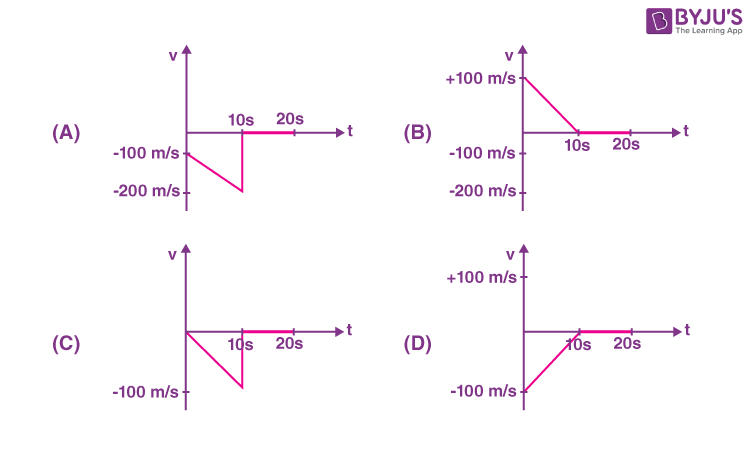Sol. |v10| = (100 + 10 × 10) m/s

v10 = – 200 m/s and v0 = – 100 m/s

from 10s to 20s velocity remains zero

⇒ from t = 0 s to 10 s velocity increases in magnitude linearly.

⇒ graph given in option A fits correctly.

3. Sand is being dropped from a stationary dropper at a rate of 0.5 kgs–1 on a conveyor belt moving with a velocity of 5 ms–l. The power needed to keep the belt moving with the same velocity will be

(A) 1.25 W

(B) 2.5 W

(C) 6.25 W

(D) 12.5 W

Sol.

$$\begin{array}{l} \frac{dm}{dt}=0.5 \text{ kg/s}\end{array}$$
$$\begin{array}{l}v = 5~ \text{m/s}\end{array}$$
$$\begin{array}{l}F = \frac{vdm}{dt} = 2.5 ~\text{kg m/s}^2\end{array}$$
$$\begin{array}{l}P=\bar{F}\cdot\bar{v} = (2.5)(5) \text{W} = 12.5~ \text{W}\end{array}$$

4. A bag is gently dropped on a conveyor belt moving at a speed of 2 m/s. The coefficient of friction between the conveyor belt and bag is 0.4. Initially the bag slips on the belt before it stops due to friction. The distance travelled by the bag on the belt during slipping motion, is

[Take g = 10 m/s2]

(A) 2 m

(B) 0.5 m

(C) 3.2 m

(D) 0.8 ms

Sol. v = 2 m/s

μ = 0.4

a = +(0.4) (g)

= + 4 m/s2

v2u2 = 2 as

⇒ (4) = 2 × (4) (s)

s = 0.5 m

5. Two cylindrical vessels of equal cross-sectional area 16 cm2 contain water upto heights 100 cm and 150 cm respectively. The vessels are interconnected so that the water levels in them become equal. The work done by the force of gravity during the process, is [Take, density of water = 103 kg/m3 and g = 10 ms–2]

(A) 0.25 J

(B) 1 J

(C) 8 J

(D) 12 J

Sol. A = 16 × 10–4 m2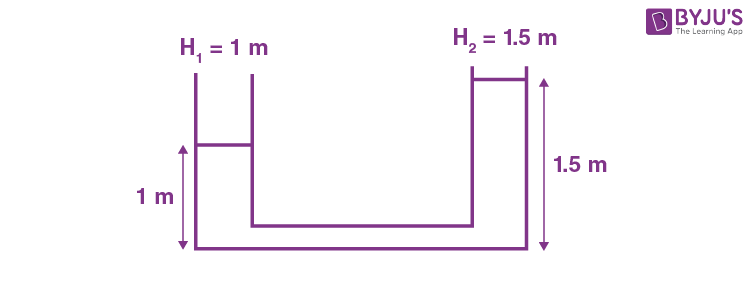$$\begin{array}{l} E_{in} = m_1g\frac{H_1}{2} + m_2g\frac{H_2}{2}\end{array}$$
$$\begin{array}{l}=\rho g\frac{A}{2}(H_1^2 + H_2^2) = \rho g\frac{A}{2}(1^2 + 1.5^2)\end{array}$$
$$\begin{array}{l}E_{\text{fin}}=\rho g\frac{A}{2}(2H^2)=\rho g\frac{A}{2}(2\times 1.25^2)\end{array}$$
$$\begin{array}{l}W=\rho g \frac{A}{2}(3.25 – 3.125) = 1\text{ J } \end{array}$$

6. Two satellites A and B, having masses in the ratio 4 : 3, are revolving in circular orbits of radii 3r and 4r respectively around the earth. The ratio of total mechanical energy of A to B is

(A) 9 : 16

(B) 16 : 9

(C) 1 : 1

(D) 4 : 3

Sol.

$$\begin{array}{l}U=-\frac{GM_em}{2r}\end{array}$$
$$\begin{array}{l}\text{So,} \frac{U_A}{U_B}=\frac{m_A}{m_B}\times \frac{r_B}{r_A}\end{array}$$
$$\begin{array}{l}=\frac{4}{3}\times\frac{4}{3}=\frac{16}{9}\end{array}$$

7. If K1 and K2 are the thermal conductivities, L1 and L2 are the lengths and A1 and A2 are the cross sectional areas of steel and copper rods respectively such that

$$\begin{array}{l}\frac{K_2}{K_1}=9, \frac{A_1}{A_2}=2, \frac{L_1}{L_2}=2\end{array}$$
. Then, for the arrangement as shown in the figure, the value of temperature T of the steel-copper junction in the steady state will be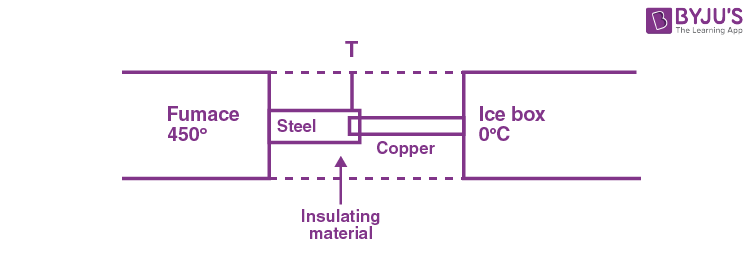(A) 18°C

(B) 14°C

(C) 45°C

(D) 150°C

Sol.

$$\begin{array}{l}450-T=\frac{dQ}{dt}\times\frac{l_1}{K_1A_1}\end{array}$$
$$\begin{array}{l}T-0=\frac{dQ}{dt}\times \frac{l_2}{K_2A_2}\end{array}$$
$$\begin{array}{l}\text{So, } \frac{450-T}{T}=\frac{K_2A_2l_1}{K_1A_1l_2}=9\times \frac{1}{2}\times 2 = 9\end{array}$$
$$\begin{array}{l}450 – T = 9T \\\Rightarrow T = 45~^\circ \text{C}\end{array}$$

A. When small temperature difference between a liquid and its surrounding is doubled, the rate of loss of heat of the liquid becomes twice.

B. Two bodies P and Q having equal surface areas are maintained at temperature 10°C and 20°C. The thermal radiation emitted in a given time by P and Q are in the ratio 1 : 1.15.

C. A carnot Engine working between 100 K and 400 K has an efficiency of 75%.

D. When small temperature difference between a liquid and its surrounding is quadrupled, the rate of loss of heat of the liquid becomes twice.

Choose the correct answer from the options given below

(A) A, B, C only

(B) A, B only

(C) A, C only

(D) B, C, D only

Sol. From Newton’s cooling law

$$\begin{array}{l}\frac{dQ}{dt}=-k(T-T_s)\end{array}$$
the statement A is correct

For B

$$\begin{array}{l}U=\sigma eAT^4\end{array}$$
$$\begin{array}{l}\text{So, }\frac{U_1}{U_2}=\left( \frac{283}{293}\right)^4 \simeq \frac{1}{1.15}\end{array}$$

Statement B is correct

For C

$$\begin{array}{l}\eta = 1 – \frac{T_1}{T_2}=1-\frac{100}{400}=\frac{3}{4}\end{array}$$

So, efficiency is 75% C is correct

For D

From Newton’s law of cooling

$$\begin{array}{l}\frac{dQ}{dt}=-k(T-T_s)\end{array}$$

The statement is wrong.

9. Same gas is filled in two vessels of the same volume at the same temperature. If the ratio of the number of molecules is 1 : 4, then

A. The r.m.s. velocity of gas molecules in two vessels will be the same.

B. The ratio of pressure in these vessels will be 1 : 4.

C. The ratio of pressure will be 1 : 1.

D. The r.m.s. velocity of gas molecules in two vessels will be in the ratio of 1 : 4.

Choose the correct answer from the options given below.

(A) A and C only

(B) B and D only

(C) A and B only

(D) C and D only

Sol.

$$\begin{array}{l}v_{\text{rms}}=\sqrt{\frac{3RT}{M_0}}\end{array}$$
because T is same

vrms will be same so, A is correct D is incorrect

$$\begin{array}{l}\frac{P_1}{P_2}=\frac{n_1RT_1/V_1}{n_2RT_2/V_2}=\frac{n_1}{n_2}=\frac{1}{4}\end{array}$$

B is correct

C is incorrect

10. Two identical positive charges Q each are fixed at a distance of ‘2a’ apart from each other. Another point charge q0 with mass ‘m’ is placed at midpoint between two fixed charges. For a small displacement along the line joining the fixed charges, the charge q0 executes SHM. The time period of oscillation of charge q0 will be

$$\begin{array}{l}(\text{A})\ \sqrt{\frac{4\pi^3\varepsilon_0 ma^3}{q_0Q}}\end{array}$$
$$\begin{array}{l}(\text{B})\ \sqrt{\frac{q_0Q}{4\pi^3\varepsilon_0 ma^3}}\end{array}$$
$$\begin{array}{l}(\text{C})\ \sqrt{\frac{2\pi^2\varepsilon_0 ma^3}{q_0Q}}\end{array}$$
$$\begin{array}{l}(\text{D})\ \sqrt{\frac{8\pi^3\varepsilon_0 ma^3}{q_0Q}}\end{array}$$

Sol.(x << a) (α is acceleration)

$$\begin{array}{l}F_{\text{net}}=-\left(\frac{kq_0Q}{(a-x)^2} – \frac{kQq_0}{(a+x)^2}\right)\end{array}$$
$$\begin{array}{l}m\alpha = -\frac{kq_0Q}{a^4}4ax\end{array}$$
$$\begin{array}{l}\Rightarrow \alpha = -\frac{4kq_0Q}{ma^3}x\end{array}$$
$$\begin{array}{l}\text{So, } T=2\pi\sqrt{\frac{4\pi \varepsilon_0 ma^3}{4q_0Q}}\end{array}$$
$$\begin{array}{l}\text{or, } T=\sqrt{\frac{4\pi^3 \varepsilon_0 ma^3}{q_0Q}}\end{array}$$

11. Two sources of equal emfs are connected in series. This combination is connected to an external resistance R. The internal resistances of the two sources are r1 and r2 (r1 > r2). If the potential difference across the source of internal resistance r1 is zero, then the value of R will be :

$$\begin{array}{l}(\text{A})\ r_1 – r_2\end{array}$$
$$\begin{array}{l}(\text{B})\ \frac{r_1r_2}{r_1+r_2}\end{array}$$
$$\begin{array}{l}(\text{C})\ \frac{r_1+r_2}{2}\end{array}$$
$$\begin{array}{l}(\text{D})\ r_2 – r_1\end{array}$$

Sol.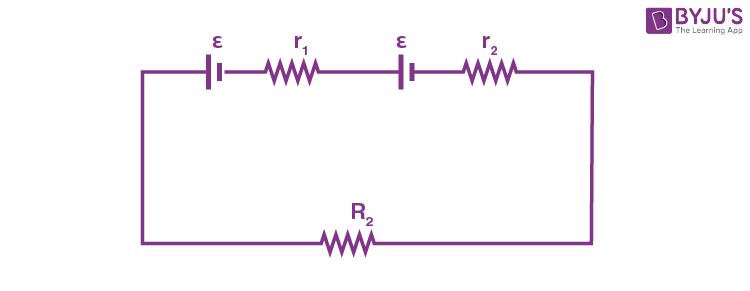$$\begin{array}{l}\Delta V = 0 \Rightarrow \frac{2\varepsilon}{r_1 + r_2 + R}r_1= \varepsilon\end{array}$$
$$\begin{array}{l}\Rightarrow R = r_1 – r_2\end{array}$$

12. Two bar magnets oscillate in a horizontal plane in earth’s magnetic field with time periods of 3 s and
4 s respectively. If their moments of inertia are in the ratio of 3 : 2, then the ratio of their magnetic moments will be :

(A) 2 : 1

(B) 8 : 3

(C) 1 : 3

(D) 27 : 16

Sol.

$$\begin{array}{l}T=2\pi \sqrt{\frac{I}{MB_H}}\end{array}$$
$$\begin{array}{l}\Rightarrow \frac{T_1}{T_2}=\sqrt{\frac{I_1}{I_2}}\sqrt{\frac{M_2}{M_1}}\end{array}$$
$$\begin{array}{l}\Rightarrow \frac{3}{4} = \sqrt{\frac{3}{2}} \sqrt{\frac{M_2}{M_1}}\end{array}$$
$$\begin{array}{l}\frac{M_1}{M_2} = \frac{3}{2}\times \frac{16}{9}=\frac{8}{3}\end{array}$$

13. A magnet hung at 45° with magnetic meridian makes an angle of 60° with the horizontal. The actual value of the angle of dip is

$$\begin{array}{l}(\text{A})\ \tan^{-1}\left(\sqrt{\frac{3}{2}}\right)\end{array}$$
$$\begin{array}{l}(\text{B})\ \tan^{-1}\left( \sqrt{6}\right)\end{array}$$
$$\begin{array}{l}(\text{C})\ \tan^{-1}\left( \sqrt{\frac{2}{3}}\right)\end{array}$$
$$\begin{array}{l}(\text{D})\ \tan^{-1}\left( \sqrt{\frac{1}{2}}\right)\end{array}$$

Sol.

$$\begin{array}{l}\tan 60^{\circ} = \frac{B_0 \sin \delta}{B_0 \cos \delta \cos 45 ^{\circ}}\end{array}$$
$$\begin{array}{l}\Rightarrow \tan \delta = \sqrt{\frac{3}{2}}\end{array}$$
$$\begin{array}{l}\Rightarrow \delta = \tan^{-1} \left( \sqrt{\frac{3}{2}}\right)\end{array}$$

14. A direct current of 4 A and an alternating current of peak value 4 A flow through resistance of 3 Ω and 2 Ω respectively. The ratio of heat produced in the two resistances in same interval of time will be :

(A) 3 : 2

(B) 3 : 1

(C) 3 : 4

(D) 4 : 3

Sol.

$$\begin{array}{l}\text{Ratio} = \frac{i_ 1^2 R_1}{\left(\frac{i_2}{\sqrt{2}}\right)^2 R_2} = \frac{4^2\times3}{\left(\frac{4}{\sqrt{2}}\right)^2\times2}\end{array}$$

⇒ Ratio = 3 : 1

15. A beam of light travelling along X-axis is described by the electric field Ey = 900sin ω(tx/c). The ratio of electric force to magnetic force on a charge q moving along Y-axis with a speed of 3 × 107 ms–1 will be :

(Given speed of light = 3 × 108 ms–1)

(A) 1 : 1

(B) 1 : 10

(C) 10 : 1

(D) 1 : 2

Sol.

$$\begin{array}{l}\text{Ratio } = \frac {|q \overrightarrow E|}{|q \overrightarrow v \times \overrightarrow B|}\end{array}$$
$$\begin{array}{l}=\frac{E}{vB}=\frac{v_{\text{wave}}}{v}\end{array}$$
$$\begin{array}{l}\Rightarrow \text{Ratio } = \frac{3\times 10^8}{3 \times 10^7}=10\end{array}$$

16. A microscope was initially placed in air (refractive index 1). It is then immersed in oil (refractive index 2). For a light whose wavelength in air is λ, calculate the change of microscope’s resolving power due to oil and choose the correct option.

(A) Resolving power will be

$$\begin{array}{l}\frac{1}{4}\end{array}$$
in the oil than it was in the air.

(B) Resolving power will be twice in the oil than it was in the air.

(C) Resolving power will be four times in the oil than it was in the air.

(D) Resolving power will be

$$\begin{array}{l}\frac{1}{2}\end{array}$$
in the oil than it was in the air.

Sol.

$$\begin{array}{l}\because \text{ Resolving power } = \frac{2\mu \sin \theta}{1.22 \lambda} \end{array}$$
$$\begin{array}{l}\frac{P_1}{P_2}=\frac{\mu_1}{\mu_2}\times \frac{\mu_1}{\mu_2}\end{array}$$
$$\begin{array}{l}=\left( \frac{\mu_1}{\mu_2}\right)^2\end{array}$$
$$\begin{array}{l}\Rightarrow \frac{P_1}{P_2}=\frac{1}{4}\\\Rightarrow P_2 = 4P_1 \end{array}$$

17. An electron (mass m) with an initial velocity

$$\begin{array}{l}\vec{v}=v_0 \hat{i}(v_0>0)\end{array}$$
is moving in an electric field
$$\begin{array}{l}\vec{E}=E_0\hat{i}(E_0 >0)\end{array}$$
where E0 is constant. If at t = 0 de Broglie wavelength is
$$\begin{array}{l}\lambda_0 = \frac{h}{mv_0}\end{array}$$
, then its de Broglie wavelength after time t is given by

$$\begin{array}{l}(\text{A})\ \lambda_0\end{array}$$
$$\begin{array}{l}(\text{B})\ \lambda_0\left( 1 + \frac{eE_0t}{mv_0}\right)\end{array}$$
$$\begin{array}{l}(\text{A})\ \lambda_0t\end{array}$$
$$\begin{array}{l}(\text{D})\ \frac{\lambda_0}{\left( 1 + \frac{eE_0t}{mv_0}\right)}\end{array}$$

Sol.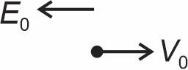$$\begin{array}{l}\therefore a_x = \frac{eE_0}{m}\hat{i}\end{array}$$
$$\begin{array}{l}\therefore v(t)=V_0+\frac{eE_0}{m}t\end{array}$$
$$\begin{array}{l}\therefore \frac{\lambda_0}{\lambda_2}=\frac{mv}{mV_0}=\left(1+\frac{eE_0t}{mV_0}\right)\end{array}$$
$$\begin{array}{l}\Rightarrow \lambda_2 = \frac{\lambda_0}{\left(1+\frac{eE_0t}{mV_0}\right)}\end{array}$$

18. What is the half-life period of a radioactive material if its activity drops to 1/16 th of its initial value in 30 years?

(A) 9.5 years

(B) 8.5 years

(C) 7.5 years

(D) 10.5 years

Sol.

$$\begin{array}{l}\because A = \frac{A_0}{\frac{t}{2^{T_{1/2}}}}\end{array}$$
$$\begin{array}{l}\Rightarrow 2^{\frac{t}{T_{1/2}}}=\frac{A_0}{A}=16\end{array}$$
$$\begin{array}{l}\Rightarrow \frac{t}{T_{1/2}} =4\end{array}$$
$$\begin{array}{l}\Rightarrow \frac{30}{T_{1/2}} =4\end{array}$$
$$\begin{array}{l}\Rightarrow T_{1/2} = \frac{30}{4}= 7.5~ \text{years} \end{array}$$

19. A logic gate circuit has two inputs A and B and output Y. The voltage waveforms of A, B and Y are shown below.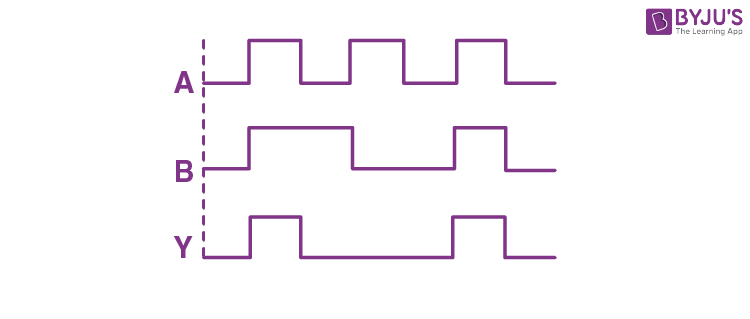The logic gate circuit is :

(A) AND gate

(B) OR gate

(C) NOR gate

(D) NAND gate

Sol. From waveforms, it is an AND gate.

20. At a particular station, the TV transmission tower has a height of 100 m. To triple its coverage range, height of the tower should be increased to

(A) 200 m

(B) 300 m

(C) 600 m

(D) 900 m

Sol.

$$\begin{array}{l}\therefore r_m = \sqrt{2Rh}\end{array}$$
$$\begin{array}{l}\Rightarrow \frac{r_1}{r_2}=\sqrt{\frac{h_1}{h_2}}\end{array}$$
$$\begin{array}{l}\Rightarrow \frac{1}{3}=\sqrt{\frac{100}{h_2}}\\\Rightarrow h_2 = 900 ~\text{m}\end{array}$$

SECTION – B

Numerical Value Type Questions: This section contains 10 questions. In Section B, attempt any five questions out of 10. The answer to each question is a NUMERICAL VALUE. For each question, enter the correct numerical value (in decimal notation, truncated/rounded-off to the second decimal place; e.g. 06.25, 07.00, –00.33, –00.30, 30.27, –27.30) using the mouse and the on-screen virtual numeric keypad in the place designated to enter the answer.

1. In a meter bridge experiment, for measuring unknown resistance ‘S’, the null point is obtained at a distance 30 cm from the left side as shown at point D. If R is 5.6 kΩ, then the value of unknown resistance ‘S’ will be ___Ω.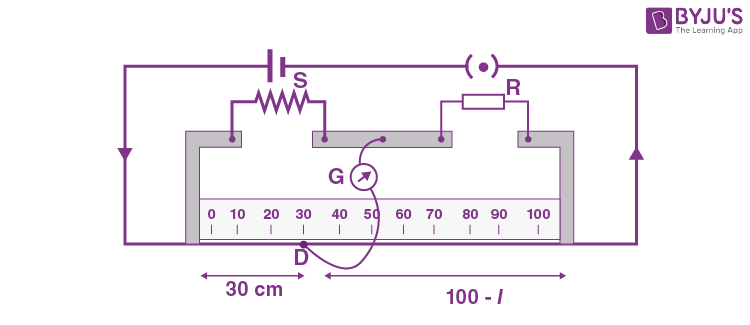Sol.

$$\begin{array}{l}\frac{R}{S}=\frac{70}{30}\end{array}$$
$$\begin{array}{l}S=\frac{3}{7}\times 5.6 \times 10^3 = 2.4 \times 10^3 \Omega = 2400 \Omega\end{array}$$

2. The one division of main scale of Vernier callipers reads 1mm and 10 divisions of Vernier scale is equal to the 9 division on main scale. When the two jaws of the instrument touch each other, the zero of the Vernier lies to the right of zero of the main scale and its fourth division coincides with a main scale division. When a spherical bob is tightly placed between the two jaws, the zero of the Vernier scale lies in between 4.1 cm and 4.2 cm and 6th Vernier division coincides with a main scale division. The diameter of the bob will be ___ × 10–2 cm.

Sol. 1 MSD = 1 mm

10 VSD = 9 MSD

$$\begin{array}{l}LC = \frac{1}{10}\ \text{mm}\end{array}$$
$$\begin{array}{l}0 + 4 \left( \frac{1}{10}\right) \text{ mm} = 0.4 \text{ mm}\end{array}$$
$$\begin{array}{l}\text{Reading } = 41 + 6 \left(\frac{1}{10}\right)\end{array}$$

= 41 + 0.6

= 41.6 mm

= 412 × 10–2 cm

3. Two beams of light having intensities I and 4I interfere to produce a fringe pattern on a screen. The phase difference between the two beams are π/2 and π/3 at points A and B, respectively. The difference between the resultant intensities at the two points is xI. The value of x will be ___.

Sol.

$$\begin{array}{l}I_{R_1} = I_1 + I_2 + 2\sqrt{I_1I_2}\cos \phi\end{array}$$
$$\begin{array}{l}I_A = I + 4I + 2 \sqrt{I\cdot 4I }\cos 90^{\circ} = 5I\end{array}$$
$$\begin{array}{l}I_B = I + 4I + 2\sqrt{I\cdot 4I}\cos 60^{\circ} = 7I\end{array}$$
$$\begin{array}{l}I_B – I_A = 2I\end{array}$$

4. To light, a W, 100 V lamp is connected, in series with a capacitor of capacitance

$$\begin{array}{l}\frac{50}{\pi \sqrt{x}} \mu F\ \text{with 200 V, 50 Hz AC source.}\end{array}$$
The value of x will be ___.

Sol.

$$\begin{array}{l}X_C = \frac{1}{wc}=\frac{\pi \sqrt{x}}{2\pi \times 50 \times 50}\times 10^6\end{array}$$
$$\begin{array}{l}v_R^2 + v_C^2 = (200)^2\end{array}$$
$$\begin{array}{l}v_C^2 = 200^2 – 100^2\end{array}$$
$$\begin{array}{l}v_C = 100\sqrt{3} V\end{array}$$
$$\begin{array}{l}v_R = 100 V\end{array}$$
$$\begin{array}{l}P =\frac{V^2}{R}\end{array}$$
$$\begin{array}{l}R=\frac{100 \times 100}{50}= 200 \ \Omega\end{array}$$
$$\begin{array}{l}i_m = \frac{1}{2}A\end{array}$$
$$\begin{array}{l}\frac{1}{2}\times x_C = 100 \sqrt{3} \Rightarrow 10^{-6}\times \frac{\sqrt{x}}{5000}\times \frac{1}{2} = 100 \sqrt{3}\end{array}$$
$$\begin{array}{l}\frac{10^{-6}\sqrt{x}}{10000 \times 100}=\sqrt{3}\end{array}$$

√x = √3

x = 3

5. A 1 m long copper wire carries a current of 1 A. If the cross section of the wire is 2.0 mm2 and the resistivity of copper is 1.7 × 10–8 Ωm, the force experienced by moving electron in the wire is ___ × 10–23N.

(Charge on electron = 1.6 × 10–19C)

Sol. I = nevdA

$$\begin{array}{l}J = \frac{E}{\rho}\end{array}$$
$$\begin{array}{l}F=eE = \frac{1.7 \times 1.6 \times 10^{-19}\times 10^{-8}}{2 \times 10^{-6}} \\= 136 \times 10^{–23} \text{N}\end{array}$$

6. A long cylindrical volume contains a uniformly distributed charge of density ρ Cm–3. The electric field inside the cylindrical volume at a distance

$$\begin{array}{l}x = \frac{2\varepsilon_0}{\rho} \text{ m}\end{array}$$
from its axis is _______ Vm–1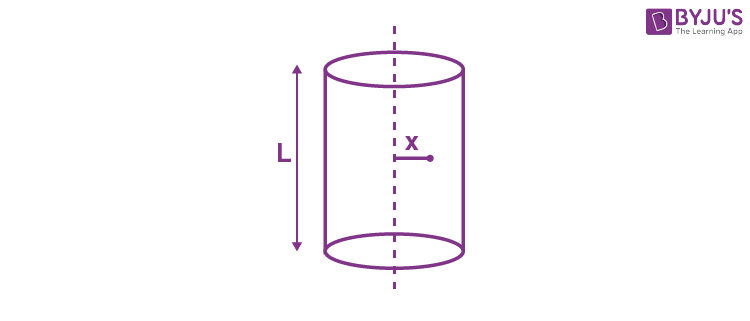Sol.

$$\begin{array}{l}E = \frac{\rho r}{2\varepsilon_0}\end{array}$$
$$\begin{array}{l}\text{at } r = \frac{2\varepsilon_0}{\rho}\end{array}$$
$$\begin{array}{l}E = \frac{\rho}{2\varepsilon_0}\left(\frac{2\varepsilon_0}{\rho}\right)\\= 1\end{array}$$

7. A mass 0.9 kg, attached a horizontal spring, executes SHM with an amplitude A1. When this mass passes through its mean position, then a smaller mass of 124 g is placed over it and both masses move together with amplitude A2. If the ratio A1/A2 is α/(α – 1), then the value of α will be _____.

Sol.

$$\begin{array}{l}(0.9)A_1\sqrt{\frac{K}{0.9}}=(0.9 + 0.124)A_2\sqrt{\frac{K}{0.9 + 0.124}}\end{array}$$
$$\begin{array}{l}\frac{A_1}{A_2}=\sqrt{\frac{0.9 + 0.124}{0.9}}\end{array}$$
$$\begin{array}{l}=\sqrt{\frac{1.024}{0.9}}\end{array}$$
$$\begin{array}{l}=\frac{\alpha}{\alpha-1}\\\alpha = 16\end{array}$$

8. A square aluminium (shear modulus is 25 × 109 Nm–2) slab of side 60 cm and thickness 15 cm is subjected to a shearing force (on its narrow face) of 18.0 × 104 N. The lower edge is riveted to the floor. The displacement of the upper edge is ______μm.

Sol.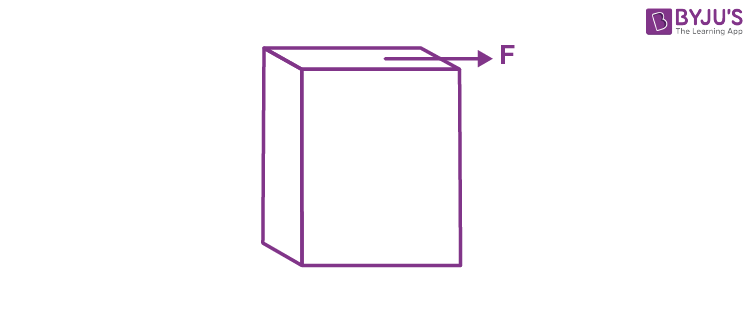$$\begin{array}{l}Y = \frac{FI}{A \Delta I}\end{array}$$
$$\begin{array}{l}\Delta I = \frac{Fl}{YA}\end{array}$$
$$\begin{array}{l}=\frac{18 \times 10^4 \times 60 \times 10^{-2}}{25 \times 10^9 \times 60 \times 15 \times 10^{-4}}\\= 48 \times 10^{-6} ~\text{m}\end{array}$$

9. A pulley of radius 1.5 m is rotated about its axis by a force F = (12t – 3t2) N applied tangentially (while t is measured in seconds). If moment of inertia of the pulley about its axis of rotation is 4.5 kg m2, the number of rotations made by the pulley before its direction of motion is reversed, will be K/π. The value of K is ________.

Sol.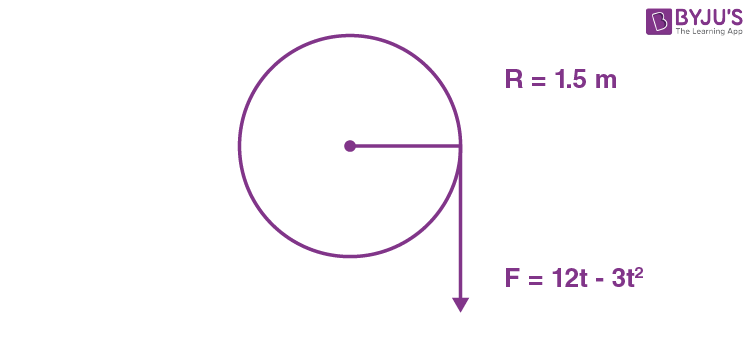I = 4.5 kg m2

$$\begin{array}{l}FR = I\alpha\end{array}$$

$$\begin{array}{l}\alpha = \frac{(12t – 3t^2)\times 1.5}{4.5}=4t-t^2\end{array}$$
$$\begin{array}{l}w=\int \alpha dt = 2t^2 – \frac{t^3}{3}\\\text{w} = 0\end{array}$$
$$\begin{array}{l}\Rightarrow t^2 \left( 2 – \frac{t}{3}\right)0\\t = 6~ \text{sec}\end{array}$$
$$\begin{array}{l}\theta = \int_{0}^{6}\left[2t^2 – \frac{t^3}{3} \right]dt = \left[\frac{2t^3}{3} – \frac{t^4}{12}\right]_0^6\end{array}$$
$$\begin{array}{l}=\left[\frac{2}{3}\times 6^3 – \frac{6^4}{12}\right]=36\end{array}$$
$$\begin{array}{l}n=\frac{36}{2\pi}\end{array}$$
$$\begin{array}{l}=\frac{18}{\pi}\end{array}$$

10. A ball of mass m is thrown vertically upward. Another ball of mass 2 m is thrown at an angle θ with the vertical. Both the balls stay in air for the same period of time. The ratio of the heights attained by the two balls respectively is 1/x. The value of x is _______.

Sol.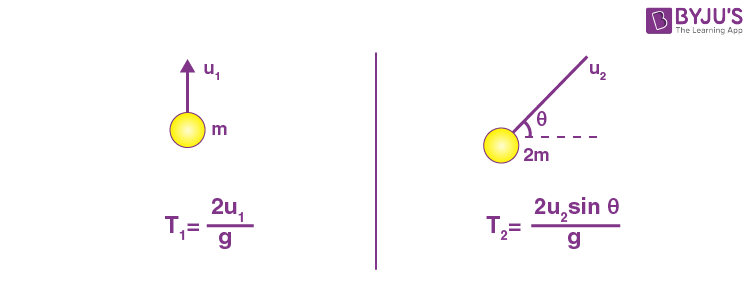u1 = u2sinθ

$$\begin{array}{l}\frac{H_1}{H_2}=\frac{\frac{u_1^2}{2g}}{u_2^2\frac{\sin^2 \theta}{2g}}\end{array}$$
$$\begin{array}{l}=\left( \frac{u_1}{u_2 \sin \theta}\right)^2= 1\end{array}$$

### Download PDF of JEE Main 2022 July 27 Shift 1 Physics Paper & Solutions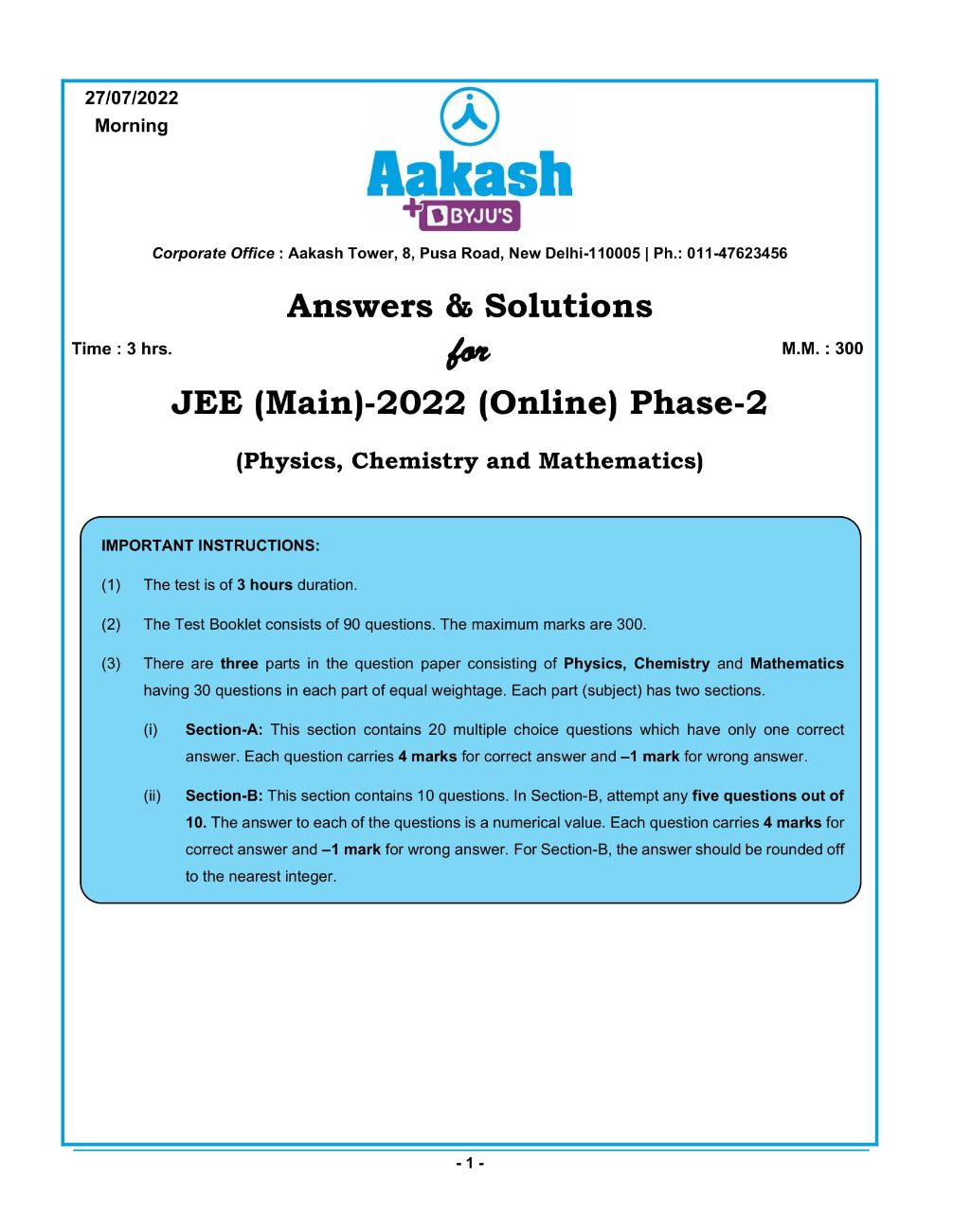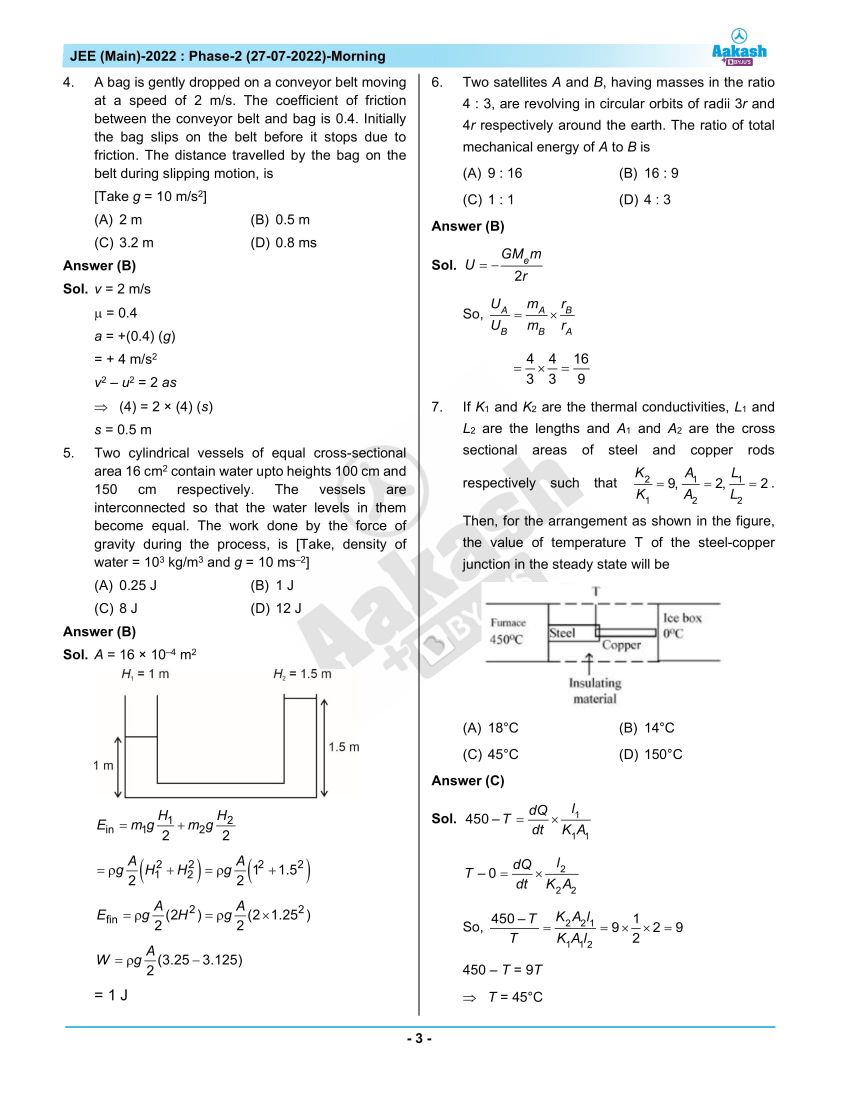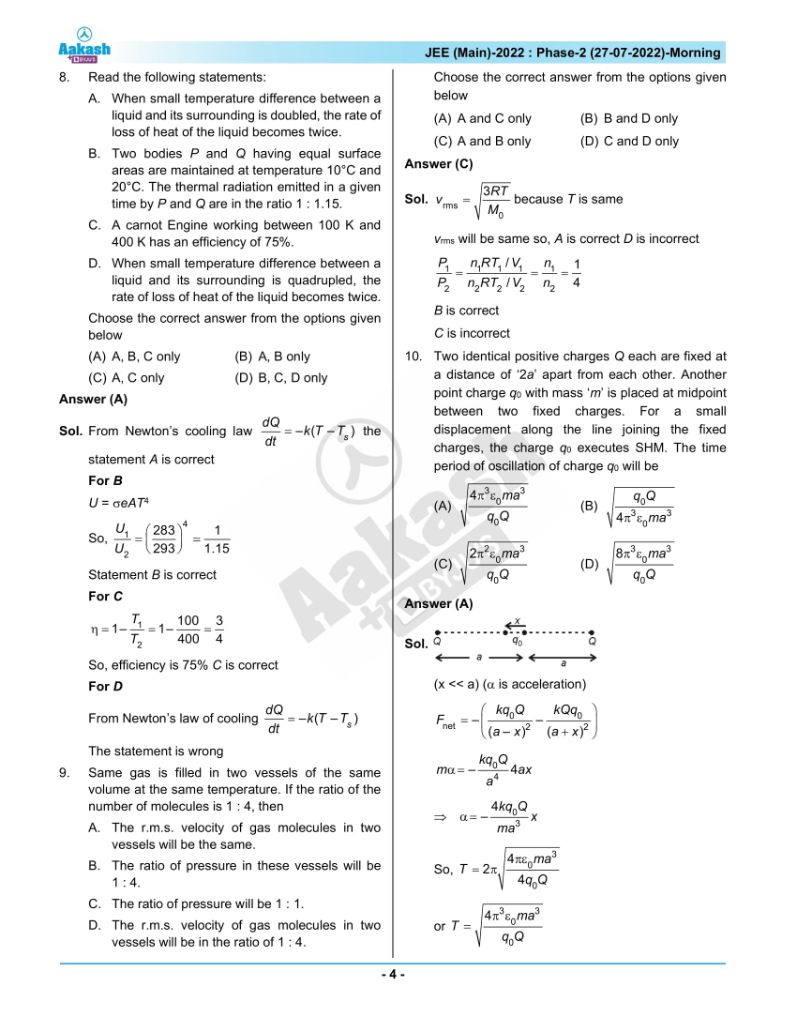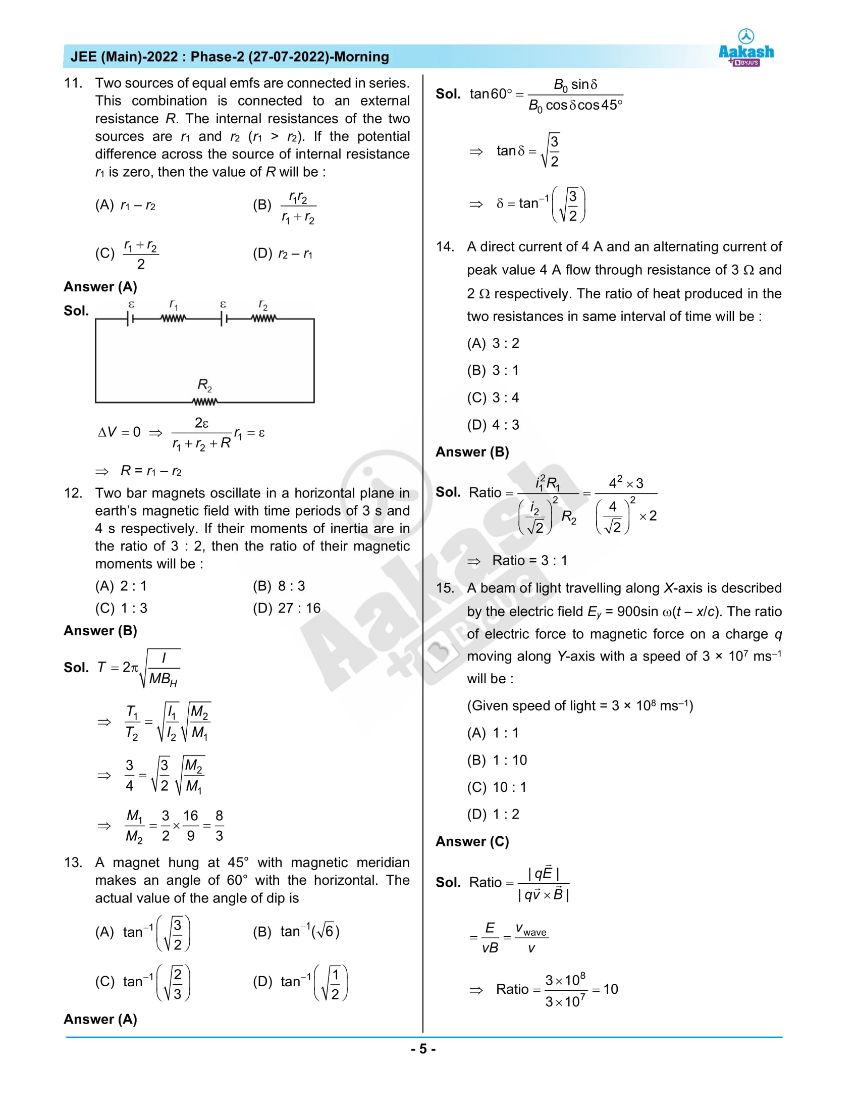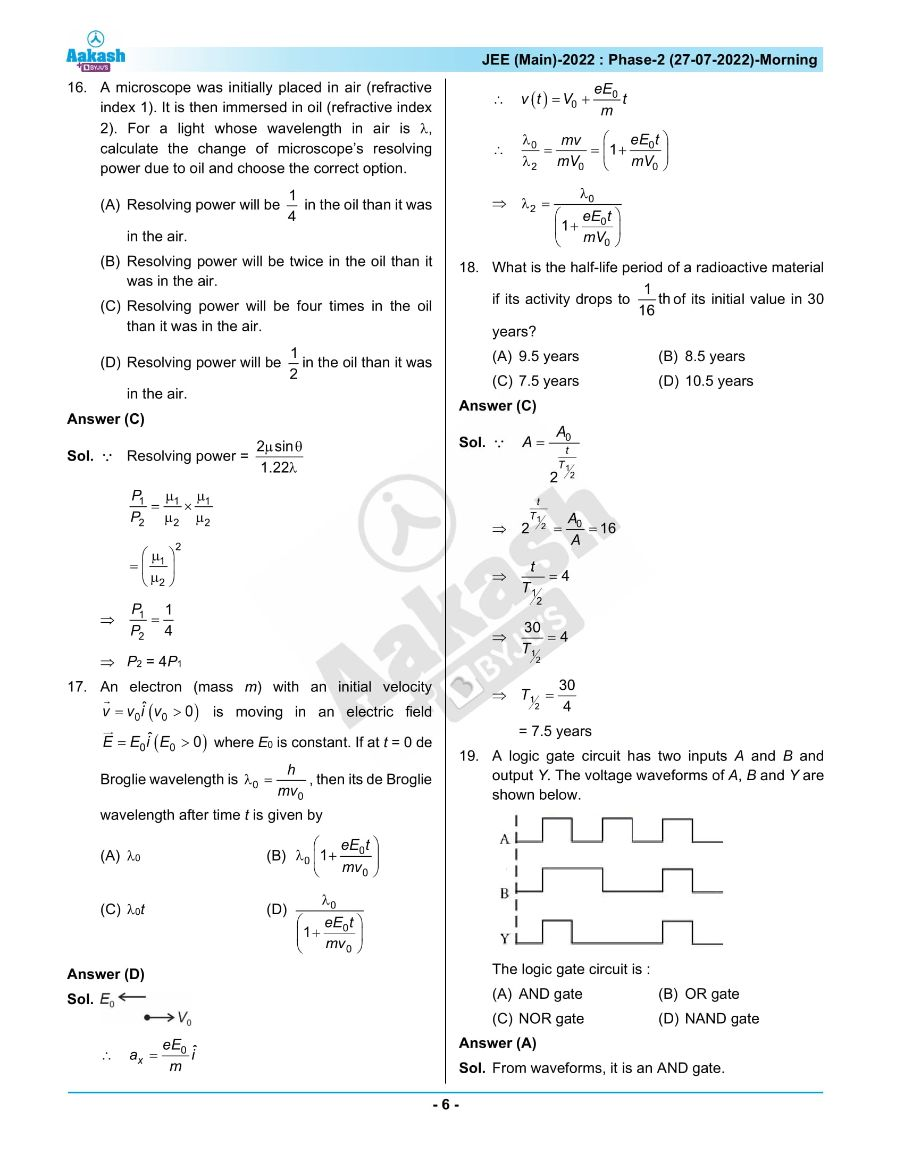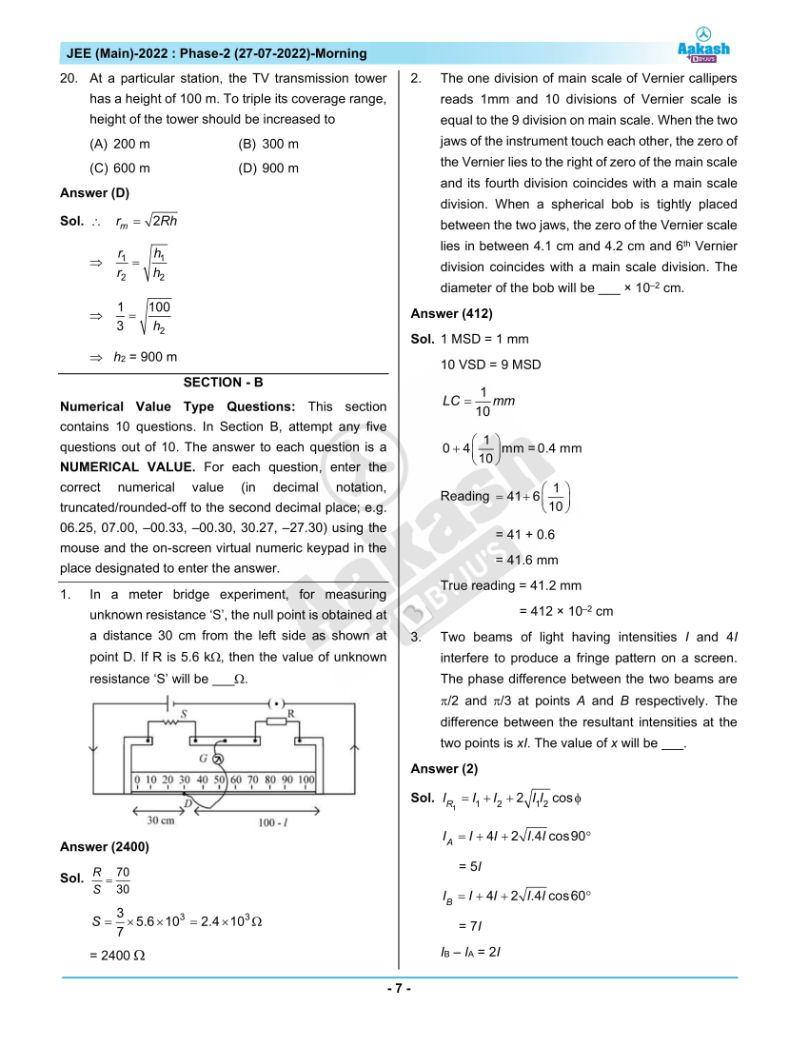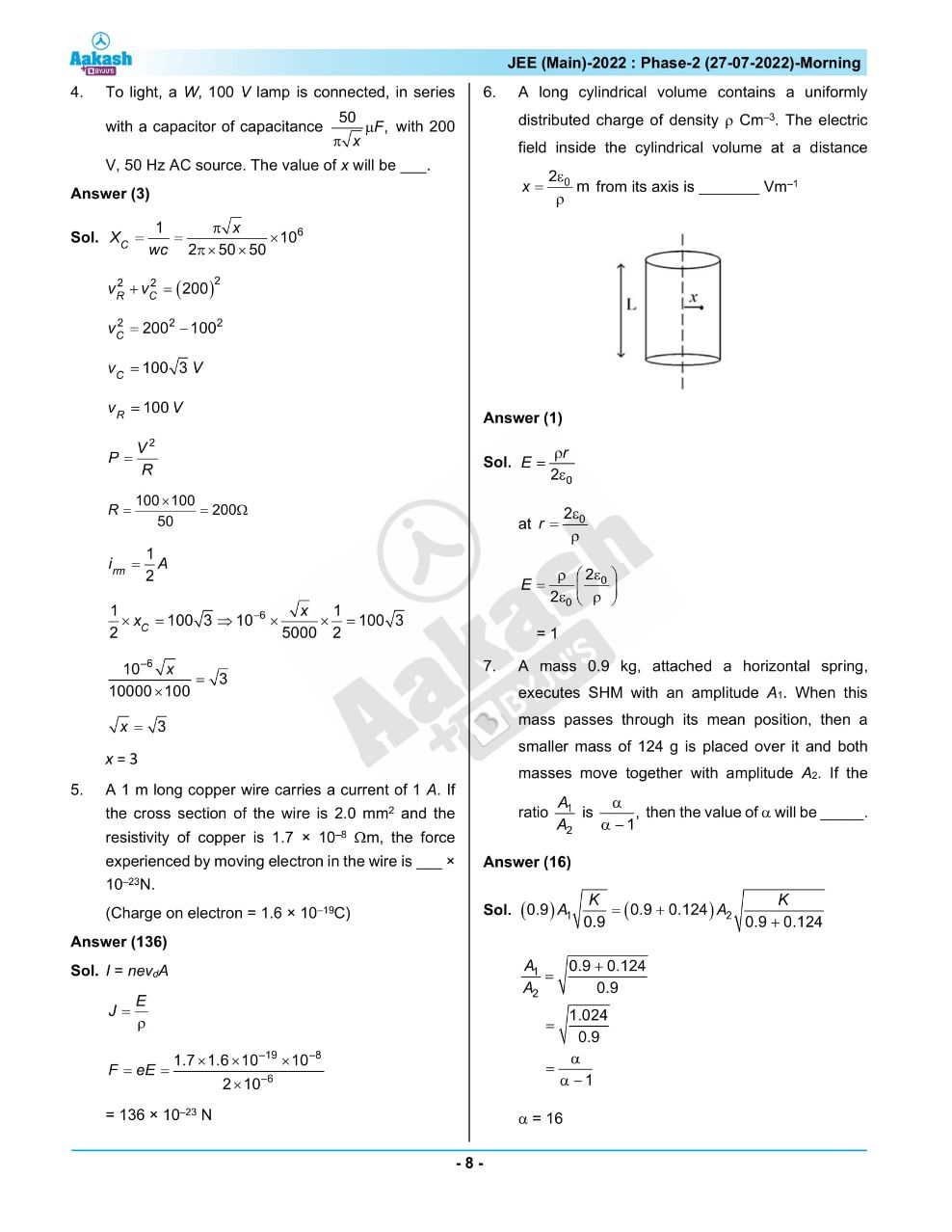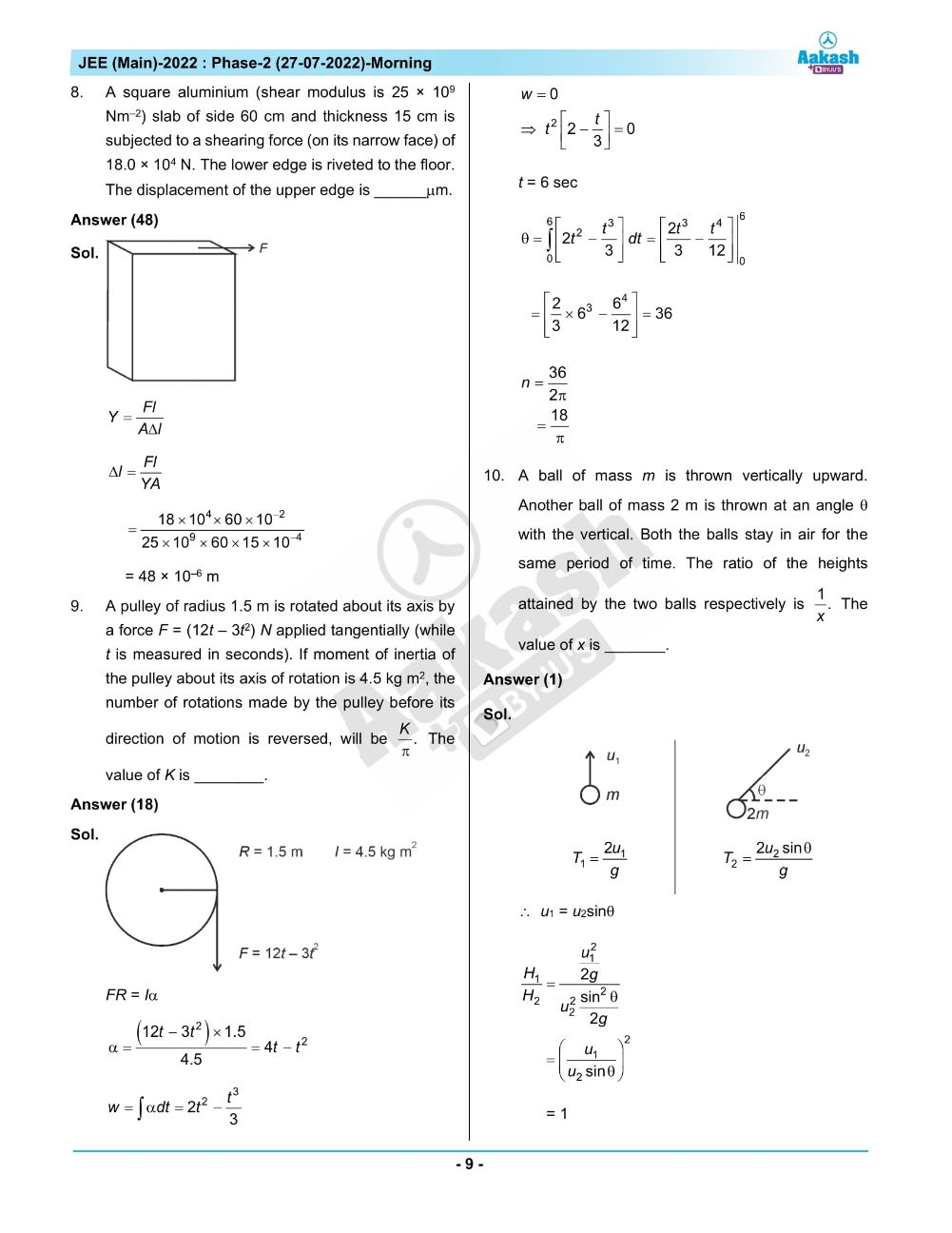## JEE Main 2022 July 27th Shift 1 Paper Analysis